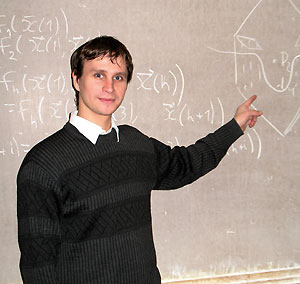[an error occurred while processing this directive][an error occurred while processing this directive][an error occurred while processing this directive]# Zhuk Dmitriy

## Ph.D., Senior researcherDmitriy was born on August 25th, 1984 in Cherepovets.

In 2001 he graduated from Vologda State School for Mathematical and Natural Sciences (VGEML) and was admitted as student to the department of Mathematics at Moscow State University. Since 2003 he studied in the subdepartment of Mathematical Theory of Intelligent Systems under the supervision of Prof. V.B.Kudryavtsev. In his thesis Dmitriy completely described all precomplete (maximal) classes in one important class of heterogenous functions.

In 2006 Dmitriy graduated with distinction from department of mathematics and continued his postgraduate studies in the subdepartment of Mathematical Theory of Intelligent Systems, where he did his research in Automata Theory.

He described different properties of definite automata and obtained different results about completeness and A-completeness problem for definite automata. Particularly, he proved that there exists continuum of closed classes of definite automata. In his PhD thesis D.Zhuk describes the precise border between algorithmically unsolvable cases and algorithmically decidable cases for A-completness problem.

Since October 2009 he holds a position of a junior researcher in the subdepartment of Mathematical Theory of Intelligent Systems and works mostly in Clone Theory. He considered the closure operator for predicates (or relations) and proposed a new technique for operating with predicates. Using this technique he obtained the following results. Dmitriy completely described the lattice of all clones of self-dual functions in three-valued logic. Thus, the class of all self-dual functions is the first maximal (or precomplete) class in three-valued logic except the class of all linear function that has such description. For every minimal clone in three-valued logic he found the cardinality of the set of all clones containing this minimal clone. He also proved that the following problem is decidable: given a finite relational structure S, decide whether S admits a near-unanimity function. This problem appeared in studying of Constraint Satisfaction Problem and was an open problem for many years.

Area of scientific interests. Automata theory, Multi-valued logic, Clone Theory, Constraint Satisfaction Problem.

Email: Íŕďčńŕňü ďčńüěî

## Publications of Dmitriy Zhuk

• Kerkhoff S., Zhuk D.N. The generation of clones with majority operations.
Algebra Universalis. May 2014.
• Zhuk D.N. The existence of a near-unanimity function is decidable
Algebra Universalis. February 2014, Volume 71, Issue 1. Pp. 31-54
• Zhuk D.N. On relations preserved by a weak near-unanimity function.
IEEE 44th International Symposium on Multiple-Valued Logic, Bremen, Germany, 2014.
• Moiseev S., Zhuk D.N. On the Clones Containing a Near-Unanimity Function.
IEEE 43rd International Symposium on Multiple-Valued Logic, Japan 2013.
• Zhuk D.N. The cardinality of the set of all clones containing a given minimal clone on three elements.
Algebra Universalis. December 2012, Volume 68, Issue 3-4. Pp 295-320.
• Zhuk D.N. The lattice of all clones of self-dual functions in three-valued logic.
2011. Preprint.
• Zhuk D.N. The cardinality of the set of all clones containing a given minimal clone on three elements.
IEEE 42st International Symposium on Multiple-Valued Logic, Canada 2012.
• Zhuk D.N. Structure of clones in the precomplete class of self-dual functions in three-valued logic.
Doklady Mathematics. Volume 83, Number 2 (2011) pp. 254-257.
• Zhuk D.N. The lattice of the clones of self-dual functions in three-valued logic.
IEEE 41st International Symposium on Multiple-Valued Logic, Finland 2011.
• Zhuk D.N. A criterion for the decidability of the A-completeness problem for definite automata
Doklady Mathematics. Volume 84, Number 1 (2011) pp. 447-449.
• Zhuk D.N. The predicate method to construct the Post lattice
Discrete Mathematics and Applications. Volume 23, Issue 2. 2011 Pp. 115128
• Zhuk D.N. The cardinality of the set of all clones containing a given minimal clone on three elements.
Algebra Universalis (Accepted).
• Zhuk D.N. The lattice of closed classes of self-dual functions in three-valued logic (Reshetka zamknutih klassov samodvoystvennih funkciy trehznachnoy logiki.)
Izdatelstvo MGU. 2011 (in Russian)
• Zhuk D.N. Cardinality of the set of all precomplete classes for definite automata.
Journal of Mathematical Sciences. Volume 169, Number 4 pp. 430-434.
• Zhuk D.N. On the classification of Post automaton bases by the decidability of the A-completeness property for definite automata
Discrete Mathematics and Applications. Volume 20, Issue 3. 2010. Pp. 337355.
• Zhuk D.N. Decidable cases of the A-completeness problem for definite automata.
Moscow, Intelligent Systems (2009) 13, 273312 (in Russian).
• Zhuk D.N. On undecidability of the completeness problem for definite automata.
Moscow, Intelligent Systems (2008) 12, 211228 (in Russian).
• Prismotrov Yu.N., Zhuk D.N. On the completeness problem in the class of automata without feedback.
Moscow, Intelligent Systems (2007) 11, 439472 (in Russian).
• Top[an error occurred while processing this directive][an error occurred while processing this directive]